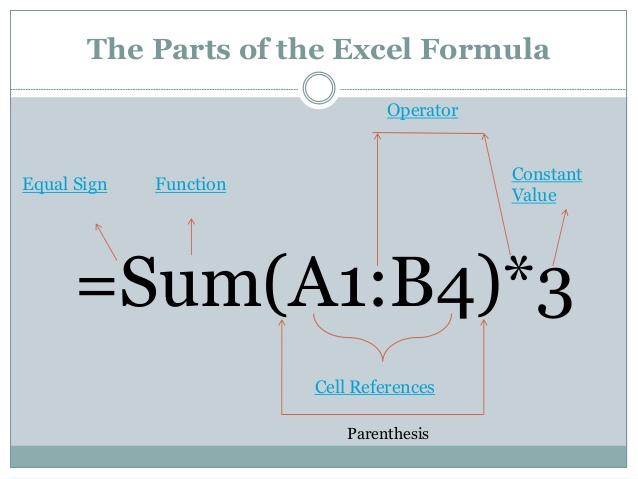# In the continuation of the series of practical tutorials for Excel software, we will provide a comprehensive tutorial on how to create and develop commonly used functions and formulas in Excel software in a visual way.

A function is a predefined formula that makes functional calculations of eigenvalues ​​in a particular statement. Excel contains several common functions that can be used to quickly obtain sum, mean, count, maximum, and minimum (MIN) values ​​for a group of cells.

In order to use functions correctly, you need to have different parts of an operation and how to create identifiers to calculate cell values ​​and references.

Note: In this tutorial, we may use operations (equivalent to function) in some places. First, watch the following video and if not enough, read on:

## Parts of a function

In order to work properly, an action must be written in a special way called syntax. The symbol that is necessary for any operation is the symbol (=) or sum (sum) and then one or more identifiers. Identifiers contain the information you want to calculate. In the following example, the values ​​from cell A1 to A20 are calculated. ID The name of the equal sign operation

## Work with IDs

Identifiers can refer to both single cells and a row of cells and must be in parentheses. You can put one or more identifiers in parentheses, depending on what type of symbol or syntax you provide for the operation. For example, the average operation from cell B1 to B9 = Average (b1: b9) will calculate the average of the values ​​from cell B1 to cell B9.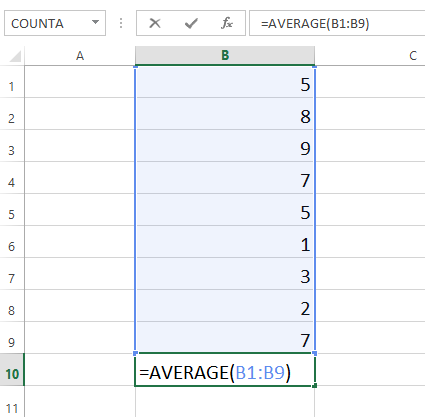Several functions must be separated by a comma (,). For example, the operation = sum (A1: A3, C1: C2, E1) sums the values ​​of all cells into three identifiers.

##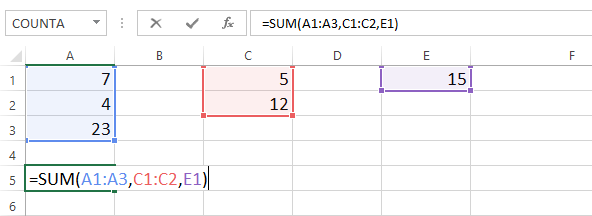Build a new function (create function)

Excel has a variety of operations available. Here are some common operations that you will use.

• SUM: This action sums the values ​​in the ID cells.
• Average: The average of the values ​​that are in the identifier, that operation calculates the sum of cells and then the sum is divided by the number and the result is obtained.
• Count: This operation counts cells numerically in the identifier. This operation is useful for quickly counting goods in a cell row.
• Max: This operation determines the maximum number of cells in an identifier.
• Min: This operation specifies the minimum value in an identifier.

## Create the main function

In our example, we will create a principal operation to calculate the average for the list of recently ordered goods with the average function.

1. Select the desired cell to perform the operation. In the example we select cell c11.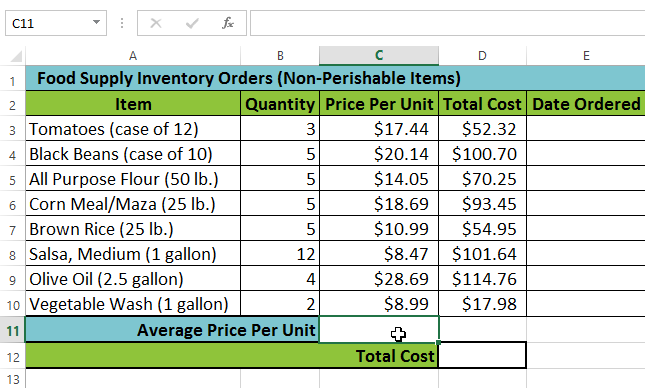2. Type the (=) sign and enter the name of the operation. You can also view the man operation from the list of suggested operations under the cell you typed. In our example, we type = = Average.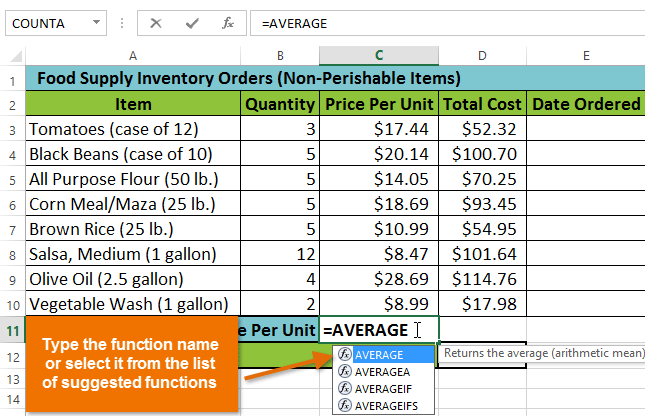3. Enter a row of cells for the ID in parentheses. In our example, (C3: C10), this formula computes the values ​​from the C10 cell, adds them, and then divides them by the total number of cells and determines the mean.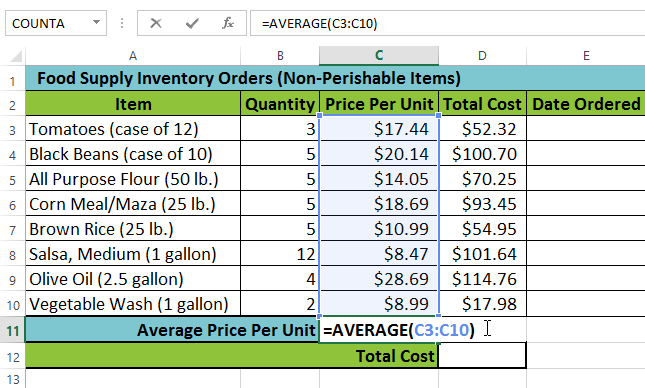4. Press the enter key on the keyboard. The operation will calculate and the result will appear in the cell. In our example, the average price per unit of goods ordered was \$ 15.93.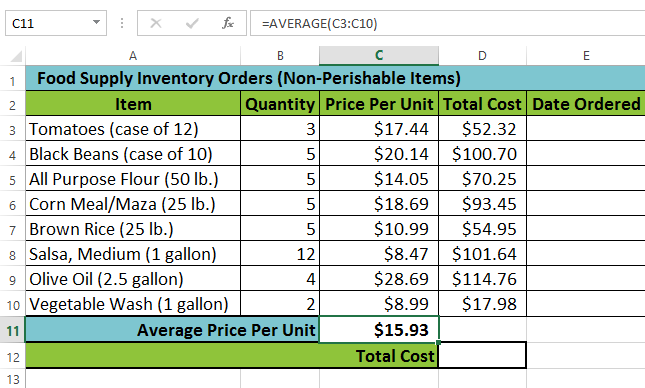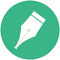Excel does not always tell you that your formula is wrong, so you should go ahead and check your formulas.

### Create an operation using the command (auto sum) (automatic sum)

The Autosam command allows you to automatically enter most common operations, including sum, average, count, minimum and maximum, into your formula.

1. Select the cell in which you want the operation to be. In the example we will select cell D12.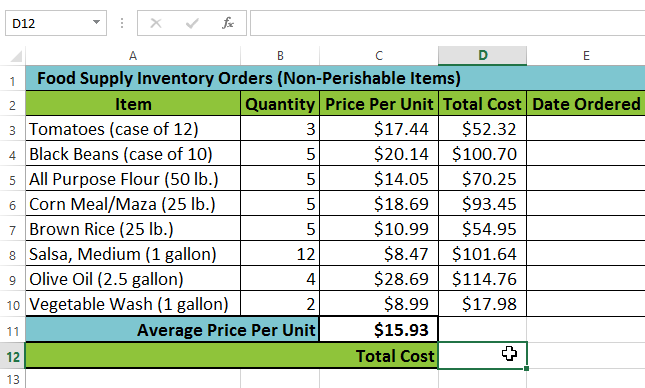2- In the Editing group, find the arrow on the home bar, then select the autosum command and then the desired operation from the menu. In our example, we choose sum.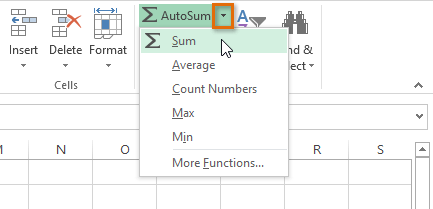3- The selected action will appear in that cell. If placed logically, the autosam command, or autocomplete, will automatically select a cell row for the identifier. In our example, cells D3 to D11 are automatically selected and their values ​​are added together to calculate the total price. You can also manually enter a row of cells into the ID.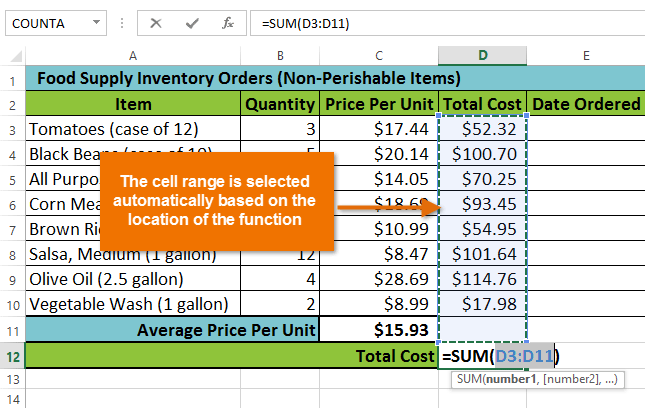4. Press the ENTER key on the keyboard. The action will calculate and the result will appear in that cell. In our example, the sum of cells D3 to D11 is \$ 606.05.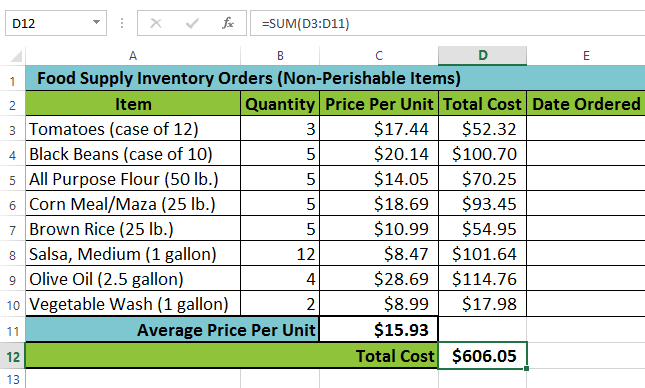The Autosam command is also accessible from the formula bar on the ribbon.

### Operations Library

While there are thousands of operations in Excel, the operations you use most often depend on the type of data contained in your workbook. You do not need to learn each operation separately, but it will be useful to consider several different types of operations when you create new files. You can search for operations by classification such as financial, logical, text, date and time, and more from the operations library on the formula bar.

• To access the operations library, select the formula bar on the ribbon.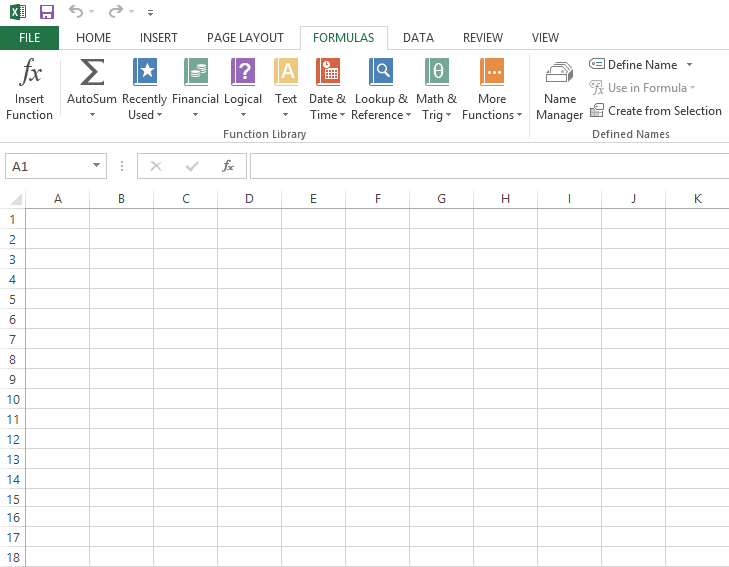• Import an operation from the operations library
• In the example below, we will use an operation to calculate the number of working days it takes for the goods to be received after being ordered. In our example, we will use the data in columns B and C to calculate the delivery time in column D.

#### 1. Select the cell in which you want the operation to be. In our example, we select cell D3.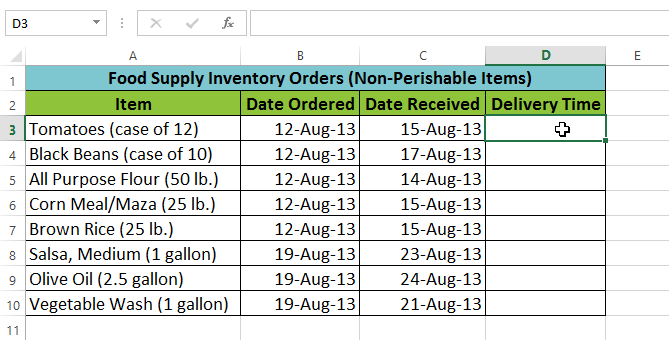2. Click the formula bar on the ribbon to access the operation.

3- From the operation group, select the operation group you want. In the example we have selected the date and time.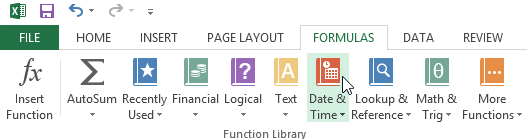4. From this option, select the operation you want. In our example, we have selected the network days operation to count the number of business days from the order date to the delivery date.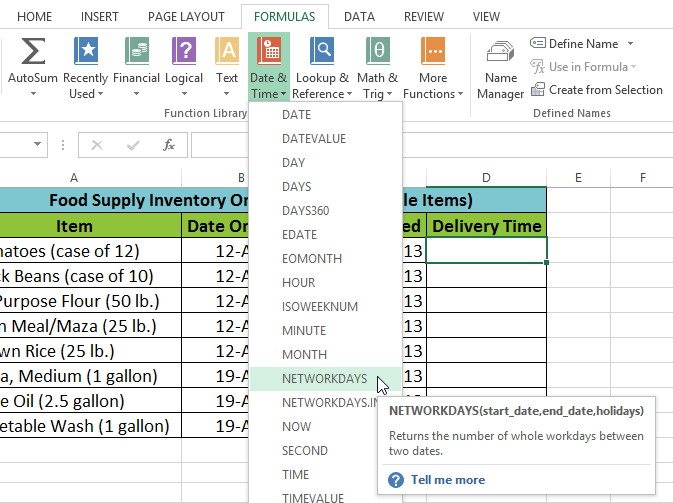5. When that option is selected, the operation ID table appears. From here you can enter or select cells that structure identifiers in operations. In our example, we have entered B3 in the start date field and C3 in the end date field…

#### 6. OK when you are satisfied with the IDs.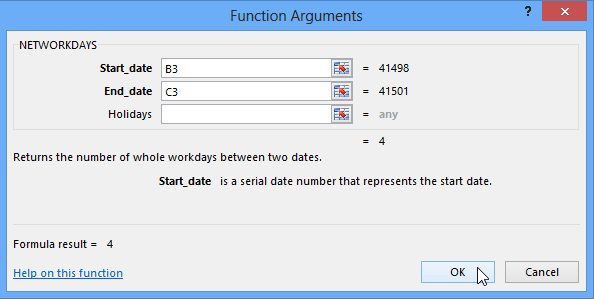7- That action is calculated and the result will appear in the cell. In our example, the result shows that it takes 4 business days to receive that order.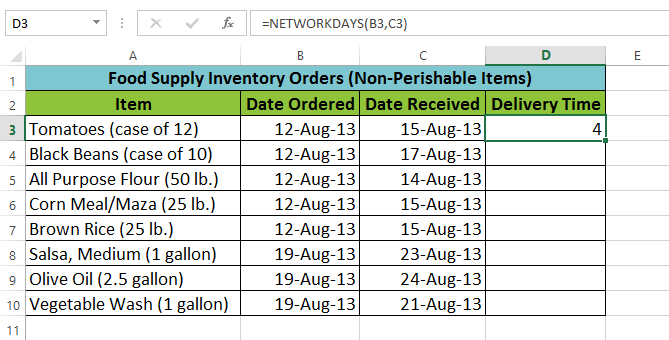Like formulas, operations can be copied to adjacent cells. To do this, move the mouse to the house where the operation is located, click. Hold and drag the handle towards the cells you want to fill. The operation will be transferred and the values ​​for those cells and their rows and columns will be calculated.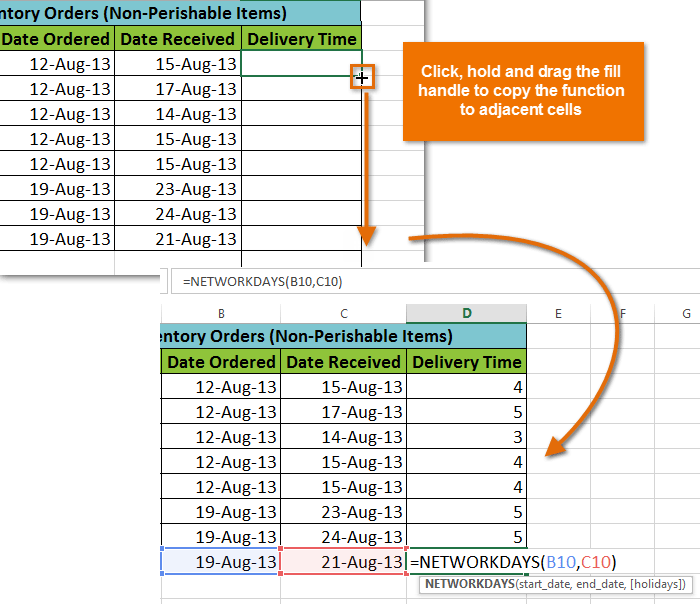Click, hold, and drag to copy a function to other cells

### Insert Operation Command

If you have trouble finding the right operation, the Insert command allows you to search by keyword for the operation. While this may be useful, it is sometimes difficult to use. If you do not have much experience in operations, you may be more successful than visiting the operations library instead. However, in a more advanced way, the Insert operation command can be a powerful way to find operations quickly.

#### Use the Insert command

In the following example, we want to find an action that counts the total number of goods ordered. We want to count the cells in the product column that use the text. We can not just use the Count operation because it only counts cells with numerical information. Instead, we look for an operation that counts the total number of cells in a row of cells.

1. Select the cell in which you want to perform the operation. In the example we have selected cell B16.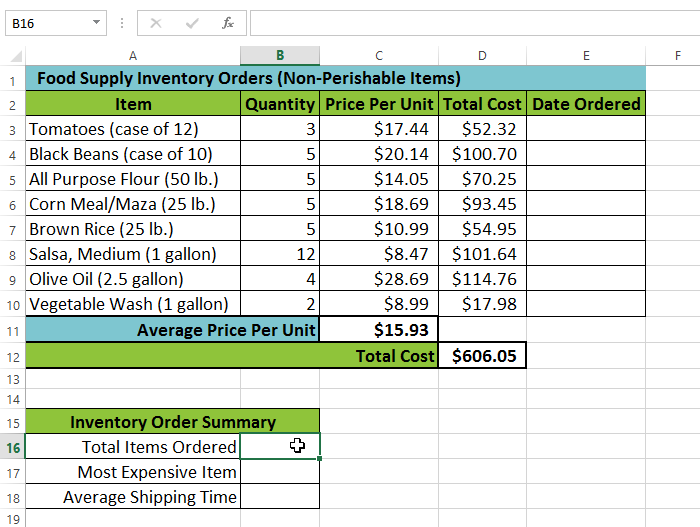2. Select the formula bar on the ribbon and then select the Insert function command.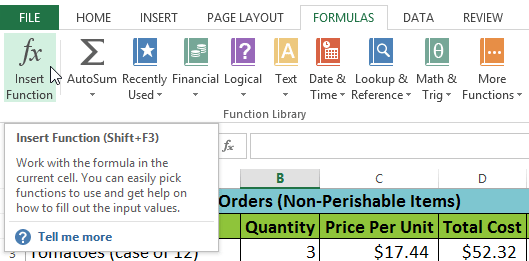3- The Insert function table will appear.

4. Type a few keywords to explain the calculation you want the action to do. Then click go. In the example we typed count cells, but you can open the drawer and search further from its subgroup.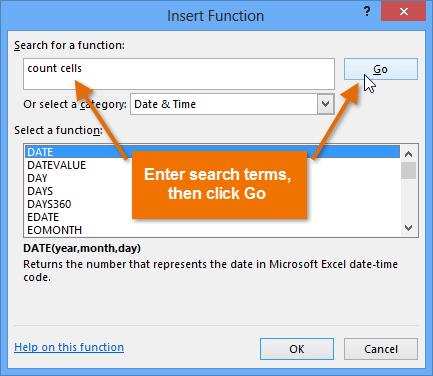5. Review the results to find the desired operation. Then click ok. In our example, we have selected count A. Because it will count the number of cells from the row of cells.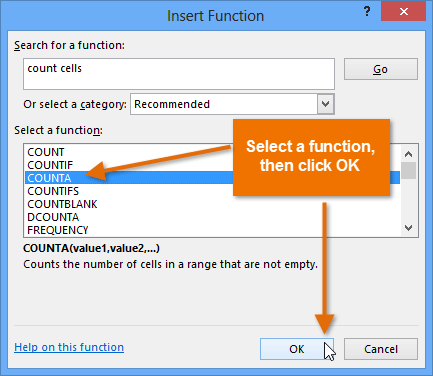6. The operational ID table will appear. Select the value 1 field. Then enter and select the desired cells. In our example, we have selected the row of cells A3: A10, (A3 to A10). You may continue to add other identifiers in the Value 2 field, but here we just want to count the number of cells A3 to A10 for us. Then click ok.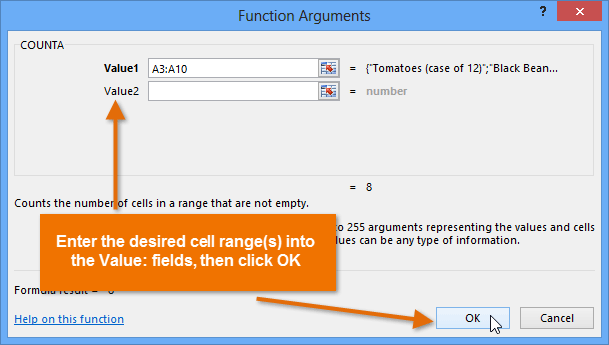7. The operation will calculate and as a result will appear in the cell. In our example, the result shows that a total of 8 products have been ordered.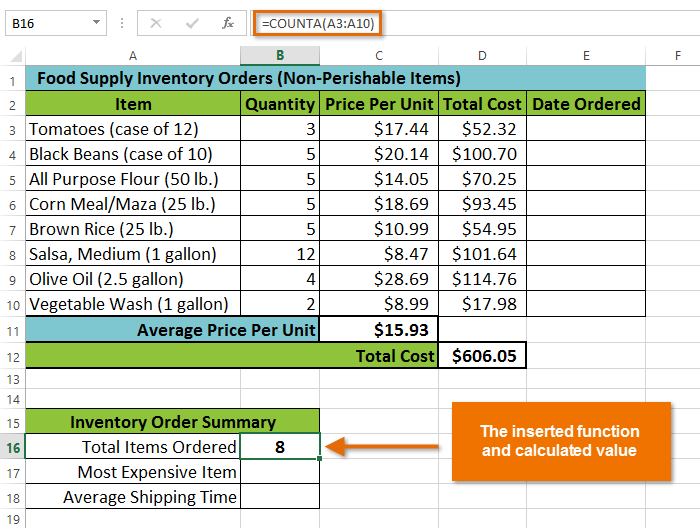If you have any questions or comments about working with Excel functions or formulas, please share them in the comments section with Quick Easy.